# Ncert Class 6 Math Fractions Exercise 7.4

Fractions Class 6 Ex. 7.4

New Ncert Class 6, Chapter 7 Math Free Solution.

Exercise 7.4

Question 1 :- Write shaded portion as fraction. Arrange them in ascending; in descending order using correct sign ‘<‘, ‘=’, ‘>’ between the fractions.

(a)(b)(c) Show 2/6;  4/6;  8/6; and 6/6; on the number line. Put appropriate-sign between the fractions given.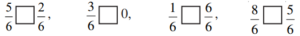Solution 1:-
(a) (i)Total number of divisions = 8
Number of shaded parts = 3
∴ Fraction = 3/8

(ii) Total number of divisions = 8
Number of shaded parts = 6
∴ Fraction = 6/8

(iii) Total number of divisions = 8
Number of shaded parts = 4
∴ Fraction = 4/8

(iv) Total number of divisions = 8
Number of shaded part = 1
∴ Fraction = 1/8

Now the fractions are :-  3/8, 6/8, 4/8 and 1/8 with same denominator.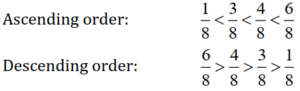(b)(i) Total number of divisions = 9
Number of shaded parts = 8
∴ Fraction = 8/9
(ii) Total number of divisions = 9
Number of shaded parts = 4
∴ Fraction = 4/9
(iii) Total number of divisions = 9
Number of shaded parts = 3
∴ Fraction = 3/9
(iv) Total number of divisions = 9
Number of shaded parts = 6
∴ Fraction = 6/9
So, fraction are 8/9, 4/9, 3/9, 6/9 with same denominator.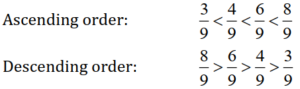(c)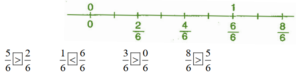Question 2 :- Compare fractions and put an appropriate sign.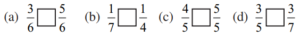Solution 2 :-

(a) Here, denominators of the two fractions are same and 3 < 5.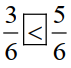(b) Here, numerators of the fractions are same and 7 < 4.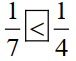(c) Here, denominators of the two fractions are same and 4 < 5.(d) Here, numerators of the two fractions are same and 5 > 7.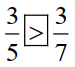Question 3 :- Make five; more such pairs and put appropriate sign.

Solution 3:-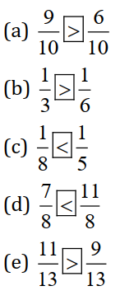Question 4 :- Look at the figures and write ’<’, or ’>’ ’=’ between the given pair of fractions.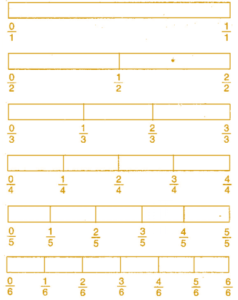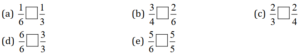Make five; more such-problems and solve them with your friends.

Solution 4 :-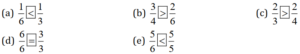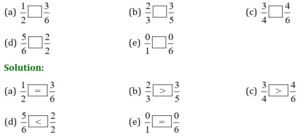Question 5 :- How-quickly can you do this? Fill appropriate sign. (‘<‘, ‘=’, ‘>’).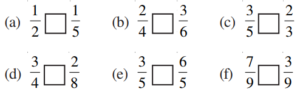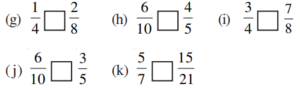Solution 5:-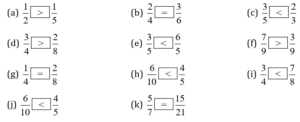Question 6 :- The following-fractions represent just three-different numbers. Separate them into three groups of equivalent-fractions; by changing each one to its simplest form.

(a) 2/12;
(b) 3/15;
(c) 8/50;
(d) 16/100;
(e) 10/60;
(f) 15/75;
(g) 12/60;
(h) 16/96;
(i) 12/75;
(j) 12/72;
(k) 3/18;
(l) 4/25;

Solution 6:-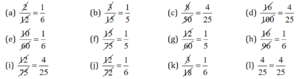Grouping the above fractions into equivalent fractions :-

I Group 1/5:-   (b), (f), (g).

II Group 1/6:- (a), (e), (h), (j), (k).

III Group 4/25:- (c), (d), (i), (l).

Question 7 :- Find answers to the following. Write and indicate how you solved them.

(a) Is 5/9 equal to 4/5 ?
(b) Is 9/16 equal to 5/9?
(c) Is 4/5 equal to 16/20 ?
(d) Is 1/15 equal to 4/30?

Solution 7:-

(a) 5/9 and 4/5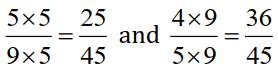[ Since, L.C.M 9 and 5 is 45]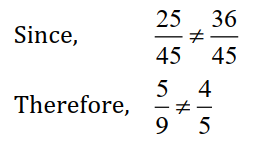(b) 9/16 and 5/9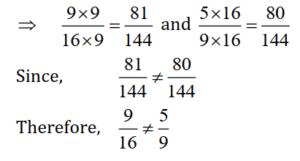[Since, L.C.M 16 and 9 is 144]

(c) 4/5 and 16/20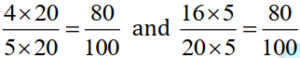[Since, L.C.M of 5 and 20 is 100]

80/100 = 80/100

4/5 = 16/20.

(d) 1/15 and 4/30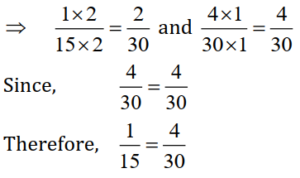Question 8 :- Ila read 25 pages of a book containing 100 pages. Lalita read 2/5 of the same book. Who read less?
Solution 8:-
Out of 100 pages, Ila reads 25 of them.
Fraction of pages 25/100 = 1/4

Lalita reads 2/5 of the same book.
2/5 = (2×20)/(5×20) = 40/100
Since 1/4 < 2/5

Question 9 :- Rafiq exercised for 3/6 of an hour, while Rohit exercised for 3/4 of an hour. Who exercised for a longer time?
Solution 9:-
Rafiq exercised for 3/6 of an hour.
Rohit exercised for 3/4 of an hour.
Comparing 3/6 and 3/4 , we get
3×2/6×2 = 6/12 and
3×3/4×3 = 9/12
Since 6 < 9
∴ 3/4 > 3/6
Hence Rohit exercised for longer time.

Question 10 :- In a class A of 25 students, 20 passed with 60%, in another class B of 30 students, 24 passed with 60% or more marks. In which class was a greater fraction of students getting with 60% or more marks?

Solution 10:-
In class A, 20 students passed with 60% or more marks out of 25 students.
∴ Fraction of students getting 60% or more marks = 20/25 = 4/5

In class B, 24 students passed with 60% or more marks out of 30 students.
∴ Fraction of students getting 60% or more marks = 24/30 = 4/5

Comparing the two fractions, we get 4/5 = 4/5
As a result, the fractions for classes A and B are identical.